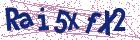# Quasi-permutation Representations of Borel and Parabolic Subgroups of Steinberg's triality groups

Document Type : Research Article

Author

Iran University of Science and Tecnology, Mazandaran

Abstract

If G is a finite linear group of degree n, that is, a finite group of automor-phisms of an n-dimensional complex vector space, or equivalently, a finite group of non-singular matrices of order n with complex coefficients, we shall say that G is a quasi-permutation group if the trace of every element of G is a non-negative rational integer. By a quasi-permutation matrix we mean a square matrix over the complex field C with non-negative integral trace.
Thus every permutation matrix over C is a quasi-permutation matrix. For a given finite group G, let c(G) denote the minimal degree of a faithful rep-resentation of G by quasi-permutation matrices over the complex numbers and let r(G) denote the minimal degree of a faithful rational valued complex character of G. The purpose of this paper is to calculate c(G) and r(G) for
the Borel and parabolic subgroups of Steinberg's triality groups.

Keywords20.1001.1.24236977.2009.2.1.1.3